Next: Fluid Closure Up: Plasma Fluid Theory Previous: Fluid Equations

# Entropy Production

It is instructive to rewrite the species-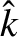energy evolution equation (222) as an entropy evolution equation. The fluid definition of entropy density, which coincides with the thermodynamic entropy density in the limit in which the distribution function approaches a Maxwellian, is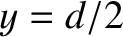(224)

The corresponding entropy flux density is written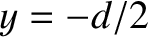(225)

Clearly, entropy is convected by the fluid flow, but is also carried by the flow of heat, in accordance with the second law of thermodynamics. After some algebra, Eq. (222) can be rearranged to give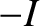(226)

where the right-hand side is given by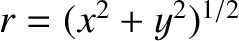(227)

It is clear, from our previous discussion of conservation laws, that the quantity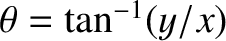can be regarded as the entropy production rate per unit volume for species. Note that entropy is produced by collisional heating, viscous heating, and heat flow down temperature gradients.Next: Fluid Closure Up: Plasma Fluid Theory Previous: Fluid Equations
Richard Fitzpatrick 2011-03-31